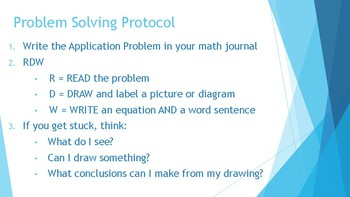# 5th Grade Eureka Math/Engage NY Application Problems: Mod. 3 (Distance Learning)Subject
File Type
Zip (3 MB|13 PPT Files)
Standards
\$3.00
• Product Description
• Standards

If you are using the Eureka Math or Engage NY math curriculum, these PowerPoints are a great way to present the daily lesson's Application Problem. This bundle is for 5th grade, Module 3, which has 16 lessons with an Application Problem for 13 of them.

Each PowerPoint has slides with a learning target and success criteria for that day's lesson, problem solving protocol following the RDW (Read, Draw, Write) format, and the Application Problem.

The Application Problem is designed to appear line by line intentionally. As I present the PPT to the class, we read and visualize each line of the problem, helping to create a better understanding. It is also useful to have students turn and talk to a neighbor about their understanding of the problem and possible ways they could solve it.

These PowerPoints can be used as whole or small group instruction, or as a rotation for your math centers and will blend perfectly with your distance learning plans. Some distance learning options include:

·Email PDF’s directly to your students. They can solve in journals or whiteboards, take pictures of their work to email back or simply send an email with their solution explaining their thinking.

·Push out via your chosen platform (Canvas, SeeSaw, etc.)

·Share slides via Screen Share during your virtual lesson. Facilitate discussions, send students to breakout rooms to discuss, note student thinking (Number Talk), or have them solve on individual whiteboards to share.

There is also a PDF that includes the solutions for each Application Problem.

If you find these PowerPoints useful, check out my other Application Problem bundles.

Note: These word problems can be used independently of Eureka/Engage NY however, the learning targets and success criteria may not make as much sense as I have based those on the goals of each entire lesson.

Solve word problems involving addition and subtraction of fractions referring to the same whole, including cases of unlike denominators, e.g., by using visual fraction models or equations to represent the problem. Use benchmark fractions and number sense of fractions to estimate mentally and assess the reasonableness of answers. For example, recognize an incorrect result 2/5 + 1/2 = 3/7, by observing that 3/7 < 1/2.
Add and subtract fractions with unlike denominators (including mixed numbers) by replacing given fractions with equivalent fractions in such a way as to produce an equivalent sum or difference of fractions with like denominators. For example, 2/3 + 5/4 = 8/12 + 15/12 = 23/12. (In general, 𝘢/𝘣 + 𝘤/𝘥 = (𝘢𝘥 + 𝘣𝘤)/𝘣𝘥.)
Total Pages
13 PPT Files